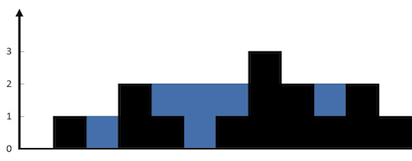# 面试题 17.21. 直方图的水量

### 题目### 题解

``````public int trap(int[] height) {
int sum = 0, length = height.length;
// 左右两边扫描大于1的值 取最小的值计算
int left = 0, right = length - 1;
// 已经访问过的最高柱子
// 向中间扫描 柱子只会越来越高
int visitedMax = 0;
while (left < right) {
int lValue = height[left], rValue = height[right];
// 左值小于当前已访问的最高柱子继续向右遍历
if (lValue <= visitedMax) {
left++;
} else if (rValue <= visitedMax) {
// 右值小于当前已访问最高柱子继续向左遍历
right--;
} else {
// 可计算体积的最高柱子为左右值中较小一个
int max = Math.min(lValue, rValue);
for (int i = left + 1; i < right; i++) {
// 只需要找到比当前柱子低的才可以接到水
if (height[i] < max) {
// 计算时需要去掉已经计算过的水数量
// 即上一次柱子的高度
sum += max - Math.max(height[i], visitedMax);
}
}
// 保存访问过最高柱子高度
visitedMax = max;
}
}

return sum;
}
``````